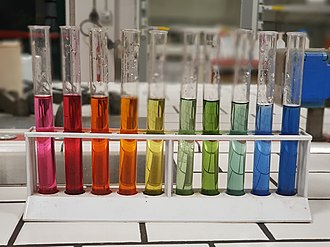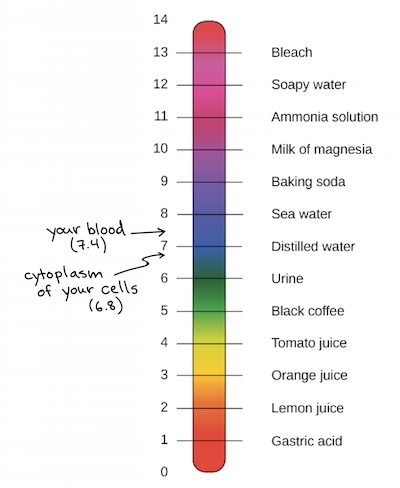# Hydroxide Ion Concentration Study Guide

INTRODUCTION

Have you ever wondered why reaction time differs for elements and why acids and bases do not react at the same rate? Some acids react vigorously, others in moderation, and some have no reaction at all. So how can we determine the strength of an acid? We figure out its pH level. Let’s talk about what pH is and the different methods you can use to find it.

### What is pH?

pH measures the hydrogen ion concentration of a solution, which helps determine its acidity or alkalinity. It ranges on a logarithmic scale from 0 to 14. If at 25° C the pH of a solution is less than 7, the solution is acidic. If the pH is greater than 7, the solution is basic. If the solution’s pH is exactly 7.0 at 25° C, it is considered ‘neutral’ because it has the same concentration of H₃O+ and OH− ions. Very strong acids and bases may have a pH value below 0 or above 14.

Many chemicals, including various liquids, do not have a pH value at all. For a solution to have a pH, it must contain water. Thus, liquids that don’t contain water, such as vegetable oil or pure alcohol, don’t have a pH value.Source

### How is pH measured?

There are several methods that can be used to determine pH. Litmus tests utilize an indicator-treated paper that changes color based on the acidity or alkalinity of a solution. Litmus paper turns red in acidic conditions, blue in basic solutions, and purple in neutral solutions. Litmus tests are limited in that they do not provide a specific pH value.

Universal pH papers are slightly more precise, changing to a wide spectrum of colors that can give you a general idea of a solution’s pH. Another method uses a mixture of indicator solutions which helps determine a pH range of 2 to 10 by changing the color of the solution.

To calculate the exact value of a solution’s pH, we use an equation proposed by Danish biochemist Søren Peter Lauritz Sørensen in 1909:

pH = -log [H+]

The log is the base-10 logarithm, and [H+] is the hydrogen ion concentration in units of moles per liter solution. Take a look at some examples of solutions with varying pHs below.Source

### What is pOH?

pOH is the measure of alkalinity, or hydroxide ion concentration, of a solution. If you know what the pH of the solution is, it gets easier to calculate its pOH value, as pH + pOH = 14. You could also apply the equation pOH = -log [OH-].

## CONCLUSION:

• pH is the measure of the hydrogen ion concentration (H₃O+) of a solution.
• pOH is the measure of alkalinity, or hydroxide ion concentration (OH-), of a solution.
• The different formulas for calculation pH and pOH are:

pOH = -log10[OH-]

[OH-] = 10 – pOH

pOH + pH = 14 for any aqueous solution

### FAQs

1. How do you find hydroxide concentration from pH?

The different formulas for calculation pOH are:

pOH = -log10[OH-]

[OH-] = 10 – pOH

pOH + pH = 14 for any aqueous solution

2. What is the hydroxide ion concentration of water?

The hydroxide ion concentration of water is 1 X 10⁻⁷ M.

We hope you enjoyed studying this lesson and learned something cool about Hydroxide Ion Concentration! Join our Discord community to get any questions you may have answered and to engage with other students just like you! Don’t forget to download our app to experience our fun VR classrooms – we promise it makes studying much more fun! 😎

## SOURCES:

1. pH Chemistry: https://byjus.com/chemistry/ph-of-acids-and-bases/. Accessed 28 Feb 2022.
2. Definition of pH in Chemistry: https://www.thoughtco.com/definition-of-ph-in-chemistry-604605. Accessed 28 Feb 2022.
3. What is pOH?:https://sciencenotes.org/what-is-poh-definition-and-calculation/. Accessed 28 Feb 2022.
4. pOH Calculations: https://www.thoughtco.com/poh-calculations-quick-review-606090. Accessed 28 Feb 2022.
5. pOH Scales and Calculations: https://www.ck12.org/c/chemistry/poh-scale-and-calculations/lesson/The-pOH-Concept-CHEM/. Accessed 28 Feb 2022.
]]>